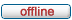# forum.alglib.net

ALGLIB forum
 It is currently Sun Sep 24, 2023 9:58 am

 All times are UTC

### Forum rules

1. This forum can be used for discussion of both ALGLIB-related and general numerical analysis questions
2. This forum is English-only - postings in other languages will be removed.

 Print view Previous topic | Next topic
Author Message
 Post subject: Fitting data into ODE system/modelPosted: Wed Nov 02, 2016 6:15 pmJoined: Wed Nov 02, 2016 6:11 pm
Posts: 3
Hello everybody!

I've got an Octave program that fits experimental data into a model of different ODEs. The Octave program uses the leasqr() function to fit the data, which uses the Levenberg-Marquardt-Algorithm.

My task is to reimplement this in C++, but I haven't found any library yet, which provides a similar function. I've seen that ALG provides functions to simulate an ODE system without experimental data, or fit data into a single ODE.

The ODE system look like that:

Code:
dA/dt = -k1*A
dB/dt = k1*A-k2*B-k3*B
dC/dt = k3*B-k4*C

Has anyone an idea how to solve this problem with ALG? I can't imagine that this is possible in Octave (or R), but nobody has accomplished that before in C++.

TopPost subject: Re: Fitting data into ODE system/modelPosted: Mon Nov 07, 2016 8:50 pmJoined: Wed Nov 02, 2016 6:11 pm
Posts: 3
Maybe I have to specify the problem a bit.
I want to estimate k1-k4 and I've experimental values for B and C (but no values for A).

Any idea or hint how (or if) I can accomplish this in ALG?

TopPost subject: Re: Fitting data into ODE system/modelPosted: Thu Dec 01, 2016 10:04 amJoined: Wed Nov 02, 2016 6:11 pm
Posts: 3
So I figured out a semi-nice implementation using lmmin() from the lmfit library (http://apps.jcns.fz-juelich.de/doku/sc/lmfit) as Levenberg-Marquardt solver, using alglibs ODE solver to get values for every lmmin() iteration. The full working example is attached below. I really would like to get a similar result using just alglib.

While the implementation is working fine in most cases, I discovered, that for some initial parameters (use 3 in my example below), the solver (odesolversolve()) gets stuck in an infinit loop. Using so debug code in the ode_model() function I discovered, that the solver is stuck at one timepoint.

Is this a general problem or is this based on my model/implementation? I know that the same initial parameter doesn't work for Octave as well I assume it's the first case, but the Octave program stops after some time. Is there a way to, at least, stop the solver in such a case?

Thx for the help!

Code:
#include <stdio.h>
#include "lmmin.h"
#include "libalglib/diffequations.h"

using namespace alglib;

/* Model for alglib ODE solver */
void
ode_model(const real_1d_array &x, double t, real_1d_array &dxdt, void *ptr)
{
double* k = (double*) ptr;
// Debug code:
/*printf("t = %.5f\n", t);
printf("k = %.5f\n", k);
printf("--\n");*/
dxdt = -k*x;
dxdt = k*x;
}

/* data structure to transmit arrays and fit model */
typedef
struct {
double *t; // Array of timepoints
double *x; // Array of datapoints (experimental)
double *sx; // Array of known start values
} data_struct;

/*
Evaluation of parameters.
Calls alglibs ODE solver to generate data with estimated parameters.
Returns the difference of experimental and simulated timepoints.
*/
void
evaluate_model(
const double *par, int m_dat, const void *data,
double *fvec, int *info)
{
// ODE solver routine
/* for readability, explicit type conversion */
data_struct *D;
D = (data_struct*)data;

// Initial Values for y
real_1d_array y;
y.setcontent(2,D->sx);

// Time points
real_1d_array t;
t.setcontent(m_dat/2,D->t);
double k[] = {par};

double eps = 0.000001; // Error tolerance
double h = 0.001; // Step length

ae_int_t m; // Number of result values
real_1d_array ttbl; // Array of time values
real_2d_array ytbl; // Matrix of result data

odesolverreport rep;
odesolverstate stt;
odesolverrkck(y, t, eps, h, stt);

odesolversolve(stt, ode_model, &k);
odesolverresults(stt, m, ttbl, ytbl, rep);

// calculate difference to experimental data
for (int i = 0; i < m_dat/2; i++ ) {
fvec[i] = D->x[i] - ytbl[i];
fvec[i+m_dat/2] = D->x[i+m_dat/2] - ytbl[i];
}
}

int
main()
{
// Array of initial parameter guess
double par[] = {0.1}; // Use 3 as initial guess for strange behaviour
// Number of parameters to estimate
int n_par = (int)(sizeof(par)/sizeof(*par));

// Array of starting values
double sx = {10,0};
// Array of time values
double t  = {0,1,2,3,4,5,6,7,8,9,10};

// Number of datapoints
int m_dat = (int)(sizeof(t)/sizeof(*t))*(sizeof(sx)/sizeof(*sx));

// Array of testdata
double x = {10,8.24,6.09,4.95,3.95,2.65,2.08,1.91,1.26,1.03,0.91,0,2.26,4.05,5.49,6.19,7.44,8.46,8.43,9.05,9.13,8.41};

// Data struct to send data to lmmin
data_struct data = { t, x, sx };

// Initialization of lmmin parameters
lm_status_struct status;
lm_control_struct control = lm_control_double;

control.verbosity = 0; // No output during parameter estimation

// perform the fit
lmmin( n_par, par, m_dat, (const void*) &data,
evaluate_model, &control, &status );

/* print results */
printf( "\nResults:\n" );
printf( "status after %d function evaluations:\n  %i = %s\n",
status.nfev, status.outcome, lm_infmsg[status.outcome] );

printf("obtained parameters:\n");
int i;
for ( i=0; i<n_par; ++i )
printf("  par[%i] = %12g\n", i, par[i]);
printf("obtained norm:\n  %12g\n", status.fnorm );

return 0;
}

TopDisplay posts from previous: All posts1 day7 days2 weeks1 month3 months6 months1 year Sort by AuthorPost timeSubject AscendingDescending

 All times are UTC

#### Who is online

Users browsing this forum: No registered users and 2 guests

 You cannot post new topics in this forumYou cannot reply to topics in this forumYou cannot edit your posts in this forumYou cannot delete your posts in this forumYou cannot post attachments in this forum

Search for: